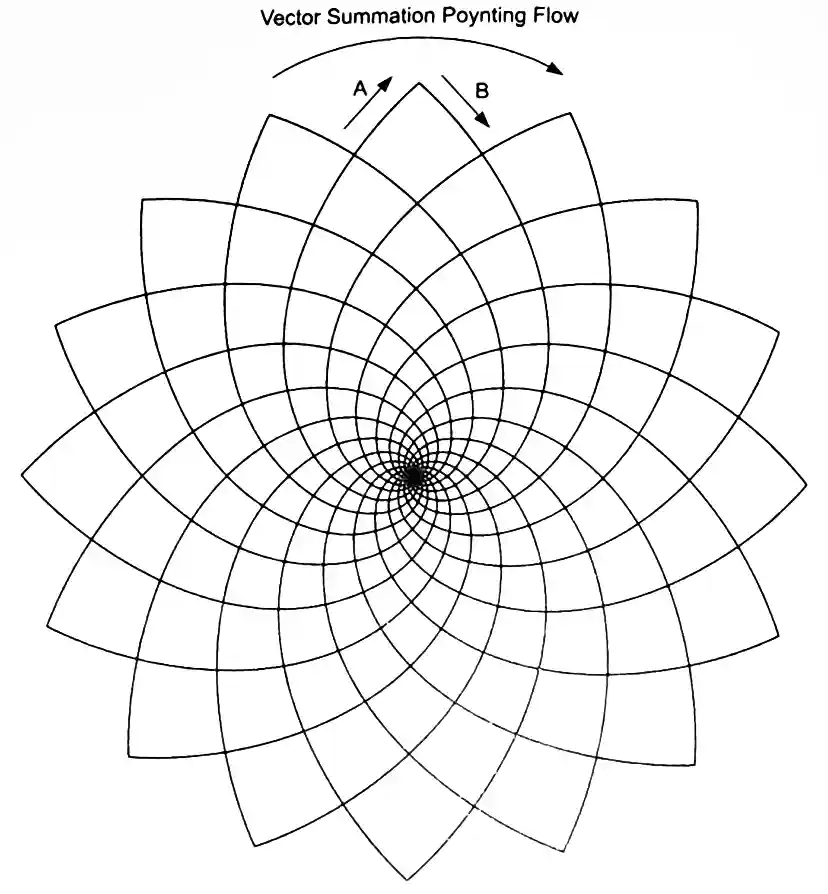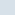The Poynting Vector of an Electron

UPDATE: July 5, 2020, One objection some people have with a classical description of an electron is that some portions of a classical electron must spin faster than light which would be impossible. The constant "c" which is used to represent the speed of light, is representing the ratio of distance traveled through space divided by duration traveled through time. What people don't realize is that at this subatomic level, it is the motion through space that causes the motion through time in the first place. In flat spacetime, the constant "c" just represents the ratio of how much of each is traversed at the same time. So if that stuff the electron is made of is spinning faster or slower through space, it spins faster or slower through time as well. The constant "c" stays the same regardless.

In mathematics, a vector describes a magnitude and a direction.

See reference: http://en.wikipedia.org/wiki/Vector_(spatial)

Electromagnetic energy propagates at the speed of light in a vacuum. The Poynting vector describes the magnitude of electrical power (electromagnetic energy per unit of time) moving through an area of space and its direction of propagation.

There is energy stored in the electromagnetic field of charged particles like the electron.

Whenever a source of an electric field, like an electron, moves in relation to anything else then there is a magnetic field that manifests and this magnetic field is at right angles to the electric field and to the direction of motion of the electric field source.

It is the effects of relative motion described in Einstien's theory of special relativity that distort the direction of the electric field to create what is perceived as the magnetic field. A charge does not have a portion of energy stored in the electric field and then more energy stored in the magnetic field. It is all the same field energy that is only perceived differently when there is relative motion.

When there is relative motion between charges, the effects of special relativity are such that it is as if there is a change in the amount of electric charge per unit length or you could say a change in the amount of electric charge per unit of time. The magnitude and direction of the field of this perceived different amount of electric charge is what is perceived as a magnetic field.

When an electron moves rapidly back and forth, its wiggling electric field radiates out into space along with the magnetic field changes that are at right angles to the electric field. This is considered an electromagnetic wave with electric and magnetic field components.

The Poynting vector points in the direction that this electromagnetic energy is radiating and its magnitude describes how much electromagnetic power (energy/time) is moving in that direction.

Even a static electromagnetic field can have a Poynting vector associated with it. For example, a loop of superconductive wire can be given a high negative charge and this charge can be induced to flow around the loop. It will have a Poynting vector that is in the direction of the flow and at right angles to the magnetic field setup by this flow of current. There is electromagnetic energy stored in the momentum of electric charge moving in the loop as represented by its magnetic field strength and the Poynting vector describes the direction of motion and magnitude of this flow of power (energy/time).

An electron may be described in a classical way as a whirlpool of electromagnetic energy having spin motion such that the electron has an electric field and also a magnetic field through its spin axis due to its spin motion relative to all things outside the electron. The direction of the magnetic field is at all places at right angles to the direction of spin of the electron.

This classical spin axis should not be confused with the Spin UP or Spin DOWN axis of the electron as described by quantum physicists. What a quantum physicist regards as the spin axis is actually the axis of gyroscopic precession of a classical electron. This is a very important distinction. The quantum mechanical spin axis is not similar to the classical spin axis but rather the classical axis of precession about which the electron spin axis is precessing or wobbling around like a top, like this link. Within an atom, it is the relative motion between orbital electrons and protons within the nucleus that make the protons appear to have relative motion and thus appear to have a magnetic field from the nucleus, (not to be confused with the weak magnetic field from each proton's own spin properties). It is the magnetic field from relative motion that affects the precession rates of electrons moving in their orbitals. There is constant electromagnetic energy exchanged between the nucleus and the orbital electrons. As described within this website's home page, paired electrons also radiate and absorb remnant electromagnetic waves to/from all other electrons in the universe.

When describing an electron as a whirlpool of electromagnetic energy (and not a solid object coated or filled with electric charge and not a dimensionless point requiring renormalization), it can be described as having a Poynting vector that is at right angles to the magnetic field of the electron. At all distances from the electron's center, its electromagnetic energy is moving circularly at the speed of light. This is the angular momentum of an electron and this electromagnetic energy of its fields is the energy of its mass. Actually, it is possible this circular Poynting vector is describing the vector summation of Poynting vectors of the energy spinning in towards the center and energy spinning back out from the center, and because the electron also precesses, it radiates and absorbs electromagnetic energy from this precession. It interacts with the radiated fields of all other precessing charged particles. Gravity, inertia and a hidden tendency for harmony of motions all arise from these hidden interactions.

But now we have a problem in describing what it is really that is spinning since most people don't want to use the concept of an aether. An aether's existence has been factored out of our equations that describe matter and energy and their relative motion in the universe. But could that have been a mistake?

If a person living in Texas is factored out of all equations describing the population of Texas does that make her disappear in real life? What if she is here all along and you just can't easily see her because you are using math based on misunderstanding and wrong assumptions to determine where and how to look for her? Anyone who has a problem with the concept of an aether can substitute "aether" with "that which gives space a specific value for the coefficient of magnetic permeability and a specific value for coefficient of electrostatic permittivity". There is something there establishing specific values of each.

We challenge you to think outside the quantum electrodynamic box.# The Poynting Vector of an Electron